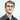# Advent of Code 2019 - Day 3

December 03, 2019

## Day 3 - Crossed Wires

### Part 1 - Hows that distance?

Find the closest point to the central port where the two wires cross.

My approach is to segment the wire into a single segment between each grid point, tracking how many steps the wire has travelled so far We can then walk these lists of segments (1 for each wire), find the ones where both wires end on the same X and Y values.

``````func spacesDirection(movement string) (string, int) {
getDirection, err := regexp.Compile("[RDUL]{1}")
check(err)
getSpaces, err2 := regexp.Compile("\\d+")
check(err2)
direction := getDirection.FindString(movement)
spacesString := getSpaces.FindString(movement)
if spacesString != "" {
spaces, err3 := strconv.Atoi(spacesString)

check(err3)
return direction, spaces
}
return "", 0
}

type Segment struct {
wire          int
startX        int
startY        int
endX          int
endY          int
previousSteps int
}

func getIntersections(segments [][]Segment) [][]Segment {
intersects := [][]Segment{}

for _, a := range segments {
for _, b := range segments {
if a.endX == b.endX && a.endY == b.endY {
intersects = append(intersects, []Segment{a, b})
}
}
}
return intersects
}

func getMinDistance(intersects [][]Segment) float64 {
minDistance := 999999.0
oX, oY := 0, 0
for _, intersect := range intersects {
distance := math.Abs(float64(intersect.endX-oX)) + math.Abs(float64(intersect.endY-oY))
if distance < minDistance && distance != 0 {
minDistance = distance
}

}
return minDistance
}
func main() {
segments := [][]Segment{[]Segment{}, []Segment{}}
for a, wire := range input {
wireMovements := strings.Split(wire, ",")
x, y, totalSteps := 0, 0, 0
for _, movement := range wireMovements {
direction, spaces := spacesDirection(movement)
for s := 1; s <= spaces; s++ {
totalSteps++
if direction == "R" {
segments[a] = append(segments[a], Segment{wire: a, startX: x, endX: x + 1, startY: y, endY: y, previousSteps: totalSteps})
x++
}
if direction == "L" {
segments[a] = append(segments[a], Segment{wire: a, startX: x, endX: x - 1, startY: y, endY: y, previousSteps: totalSteps})
x--
}
if direction == "U" {
segments[a] = append(segments[a], Segment{wire: a, startX: x, endX: x, startY: y, endY: y + 1, previousSteps: totalSteps})
y++
}
if direction == "D" {
segments[a] = append(segments[a], Segment{wire: a, startX: x, endX: x, startY: y, endY: y - 1, previousSteps: totalSteps})
y--
}

}

}
}

intersects := getIntersections(segments)

minDistance := getMinDistance(intersects)
}``````

### Part 2 - How far have I gone?

For this part we need to know how many steps the wire has moved overall before reaching the intersection

This is a small addition to the existing code above to find the smallest steps and the smallest distance.

``````func getMinStepsAndDistance(intersects [][]Segment) (int, float64) {
minDistance, minSteps := 999999.0, 999999
oX, oY := 0, 0
for _, intersect := range intersects {
distance := math.Abs(float64(intersect.endX-oX)) + math.Abs(float64(intersect.endY-oY))
steps := intersect.previousSteps + intersect.previousSteps
if distance < minDistance && steps < minSteps && distance != 0 {
minSteps = steps
minDistance = distance
}
}
return minSteps, minDistance
}``````

### Conclusion

My approach here is somewhat brute force, getIntersections is rather slow even on my modern machine as it is looping (1500000 * 1500000) times.

An alternative here that I didn’t attempt would be find intersections as we create the segments for Wire 2, potentially a lookup table for segments based on their endX,endY pair would also work as this could be created as the list of segments is added to.

If you have any feedback on my Go code, I’d be happy to hear it!

Github RepoWritten by Daniel Betteridge who lives and works in Perth, WA building useful things.